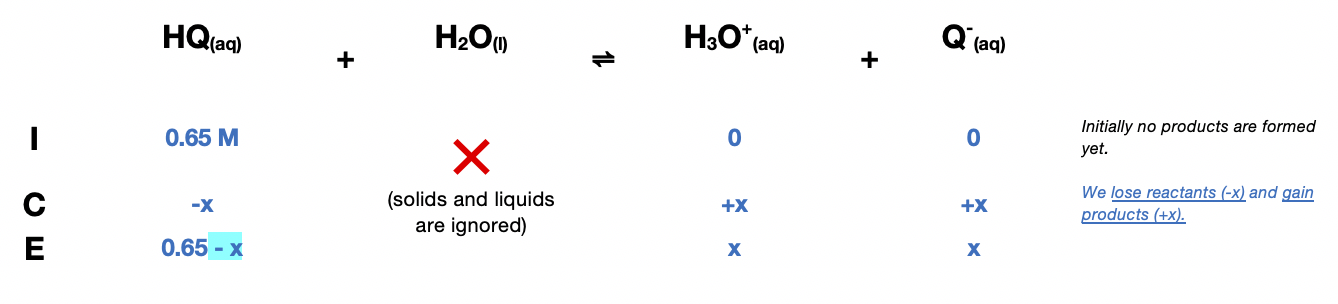# Problem: The weak acid HQ has a pKa of 4.89.(b) Calculate the [OH−] of 0.65 M HQ.

###### FREE Expert Solution

${\mathbf{K}}_{\mathbf{a}}\mathbf{=}{\mathbf{10}}^{\mathbf{-}{\mathbf{pK}}_{\mathbf{a}}}\mathbf{=}{\mathbf{10}}^{\mathbf{-}\mathbf{4}\mathbf{.}\mathbf{89}}\mathbf{=}{\mathbf{1}}{\mathbf{.}}{\mathbf{288}}{\mathbf{×}}{{\mathbf{10}}}^{\mathbf{-}\mathbf{5}}$

Construct an ICE Chart:$\overline{){{\mathbf{K}}}_{{\mathbf{a}}}{\mathbf{=}}\frac{\mathbf{products}}{\mathbf{reactants}}{\mathbf{=}}\frac{\left[{H}_{3}{O}^{+}\right]\left[{Q}^{-}\right]}{\left[\mathrm{HQ}\right]}}$

$\mathbf{1}\mathbf{.}\mathbf{288}\mathbf{×}{\mathbf{10}}^{\mathbf{-}\mathbf{5}}\mathbf{=}\frac{\left(x\right)\left(x\right)}{\mathbf{0}\mathbf{.}\mathbf{65}\mathbf{-}\mathbf{x}}$

$\mathbf{1}\mathbf{.}\mathbf{288}\mathbf{×}{\mathbf{10}}^{\mathbf{-}\mathbf{5}}\mathbf{=}\frac{{\mathbf{x}}^{\mathbf{2}}}{\mathbf{0}\mathbf{.}\mathbf{65}\mathbf{-}\overline{)\mathbf{x}}}\phantom{\rule{0ex}{0ex}}\phantom{\rule{0ex}{0ex}}\left[1.288×{10}^{-5}=\frac{{x}^{2}}{\overline{)0.65}}\right]\overline{)\left[0.65\right]}\phantom{\rule{0ex}{0ex}}\phantom{\rule{0ex}{0ex}}\sqrt{{\mathbf{x}}^{\mathbf{2}}}\mathbf{=}\sqrt{\left(1.288×{10}^{-5}\right)\left(0.65\right)}$

88% (296 ratings)###### Problem Details

The weak acid HQ has a pKa of 4.89.

(b) Calculate the [OH] of 0.65 M HQ.

Frequently Asked Questions

What scientific concept do you need to know in order to solve this problem?

Our tutors have indicated that to solve this problem you will need to apply the Weak Acids concept. If you need more Weak Acids practice, you can also practice Weak Acids practice problems.

What professor is this problem relevant for?

Based on our data, we think this problem is relevant for Professor Jing's class at GMU.

What textbook is this problem found in?

Our data indicates that this problem or a close variation was asked in Chemistry: The Molecular Nature of Matter and Change - Silberberg 8th Edition. You can also practice Chemistry: The Molecular Nature of Matter and Change - Silberberg 8th Edition practice problems.